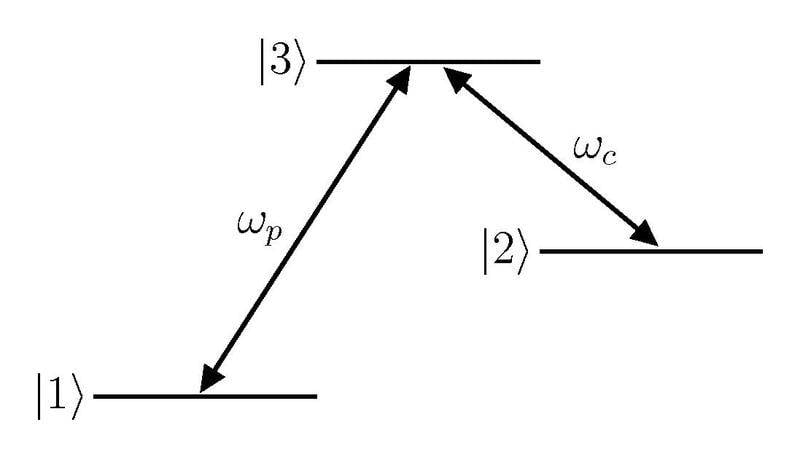Expressing the density matrix in matrix form

Homework StatementGiven the above lambda system, is it wrong to say that the density matrix is of the form ## \rho = c_1|1> + c_2|2> + c_3|3> ## ? Hence when written in matrix form (basis of ##|i>##), ## \rho ## is a diagonal matrix who's elements are the ##c_i##s?

Attachments

DrClaude
Mentor
Given the above lambda system, is it wrong to say that the density matrix is of the form ## \rho = c_1|1> + c_2|2> + c_3|3> ## ? Hence when written in matrix form (basis of ##|i>##), ## \rho ## is a diagonal matrix who's elements are the ##c_i##s?
Yes, it is wrong because that ##\rho## is not a density operator, just a quantum state (ket). For pure states, the density operator will be a projection operator,
$$| \psi \rangle \rightarrow \rho = | \psi \rangle \langle \psi |$$
In the general case, one will have (for a basis ##| i \rangle##)
$$\rho = \sum_i \sum_j c_{ij} | i \rangle \langle j|$$
with the complex coefficients ##c_{ij}## to be determined for a particular state.

Yes, it is wrong because that ##\rho## is not a density operator, just a quantum state (ket). For pure states, the density operator will be a projection operator,
$$| \psi \rangle \rightarrow \rho = | \psi \rangle \langle \psi |$$
In the general case, one will have (for a basis ##| i \rangle##)
$$\rho = \sum_i \sum_j c_{ij} | i \rangle \langle j|$$
with the complex coefficients ##c_{ij}## to be determined for a particular state.

I see okay thank you